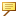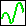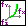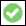> > Create a 3D parametric curve

Create a 3D parametric curve
You can create a 3D curve based on a mathematical calculation containing functional expressions such as polynomials, trigonometric functions, or exponential functions. These curves are defined by three functional expressions: fx(t), fy(t), and fz(t).
The resulting curve cannot be represented exactly, so Creo Elements/Direct Modeling generates a b-spline curve that approximates the expression. You can specify a tolerance to define the maximum distance allowed between the exact curve and the representation that is generated.
For example, you can create a variable pitch helix, as shown here:The calculation is done in millimeters (mm) and not in the units you have set in your system settings. Creo Elements/Direct Modeling cannot convert user-defined units when it approximates the curve.
To create an approximate parametric curve,
1. Click 3D Geometry and then, in the 3D Curve group, click More next toSpline 3D.
2. ClickParametric Curve Approximation in the Direct section. The Parametric Curve dialog box opens.
3. Click Part and select a part in the Structure Browser, or type a new part name. The part must be new, empty, or a wire part.
4. Change the Variable, if necessary. Any combination of normal Roman letters is allowed. Special characters, such as ü or @ are not allowed. You should use this variable in your X, Y, Z, Start, and End functions.
5. Type your X Function, Y Function, and Z Function in the appropriate fields. The function can include the following mathematical operators: +, -, *, /, sin, asin, cos, acos, atan, sqrt (square root), exp, and x^y (where y is an integer). Use normal algebraic notation, such as 10*cos(t) or 0.5*t^2.
6. Type Start and End values. These determine the interval on which the curve is defined, or the range of all values the variable will calculate.
7. Type a Tolerance, which determines how far the represented curve can be from the exact curve.
8. Clickto complete the operation.
Limitations
Logarithmic functions cannot be calculated.